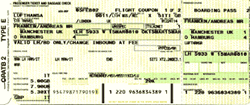#### You may also like### More Mods

What is the units digit for the number 123^(456) ?### Mod 3

Prove that if a^2+b^2 is a multiple of 3 then both a and b are multiples of 3.### Novemberish

a) A four digit number (in base 10) aabb is a perfect square. Discuss ways of systematically finding this number. (b) Prove that 11^{10}-1 is divisible by 100.

# Check Codes

##### Age 14 to 16Challenge LevelThere are many places in our lives where we meet identification numbers; for example, passports, bank accounts, credit cards, ISBN book numbers, and so on. Each identification number obeys a rule which makes it easy to check (most of the time) whether the number has been copied correctly or not and for this reason they are also called check codes. Here are some of the methods for checking the validity of these numbers. They use modulus or clock arithmetic.

Which of the following are valid identification numbers? The rules for each type are given below.

US Money Order: 123-123-123-45
Airline tickets: 123456780
Universal Product Code: 654321123456
Credit card: 1723-1001-2065-1098
ISBN number: 0 5215 3612 4
US banks: 07100013

US Postal Service Money Order : This is an eleven digit number using digits 1,2,...9 where the sum of the first ten digits is congruent to the eleventh digit modulo 9. That is $a_1a_2\cdots a_{11}$ where $a_1+ \cdots +a_{10} \equiv a_{11}$ mod 9. The last digit is called the check digit. For example 23548762912 is a valid number because the first ten digits add up to 47 which is congruent to 2 mod 9.

Airline tickets : This number can be any length. It uses the digits 0 to 9 and the last digit is a check digit. The number formed by omitting the check digit must be congruent to the check digit modulo 7. That is $a_1\cdots a_na_{n+1}$ where $a_1a_2\cdots a_n \equiv a_{n+1}$ mod 7. For example 2943739573 is a valid number because $294373957\equiv 3$ mod 7.

Universal Product Code : This is a twelve digit number $(a,b_1,b_2,b_3,b_4,b_5,c_1,c_2,c_3,c_5,c_5,d)$ using the digits 0 to 9, where
$a$ is the category of the goods (clothes, food,...),
$b_1,\ldots,b_5$ is the manufacturer's code
$c_1,\ldots,c_5$ is the code for the product,
$d$ is the check digit.
If we rewrite this type of identification code in the form $(a_1,a_2,\ldots, a_{12})$, then these codes must satisfy the following condition:

$$3(a_1+a_3+\cdots + a_{11}) + (a_2+a_4+\cdots +a_{12})\equiv 0 {\rm mod \ 10}.$$

Note that if a number $N$ is congruent to zero mod 10 it means that $N$ is a multiple of 10. For example 582039827198 is a valid code because 3(5+2+3+8+7+9)+(8+0+9+2+1+8)=130.

All Major Credit Cards : These identification codes $a_1\cdots a_{16}$ have sixteen digits using digits 0 to 9, where
$\alpha + \beta + \gamma \equiv 0$ mod 10 and where

\eqalign{\alpha &= 2(a_1 + a_3 + \cdots + a_{15}),\cr \beta &= \hbox{the number of } a_j \hbox{with } j \hbox{ odd and } a_j > 4,\cr \gamma &= a_2 + a_4 + \cdots +a_{16}.}

For example 6356 0125 0327 9616 is a valid code because $\alpha = 2(6+5+0+2+0+2+9+1)=50$, $\beta=3$, $\gamma= (3+6+1+5+3+7+6+6) = 37$ and $\alpha +\beta +\gamma = 90$.

ISBN Numbers : Books have ten digit identification numbers $a_1\cdots a_{10}$ using the digits 0 to 9 where

$$10a_1+9a_2+8a_3+\cdots +3a_8+2a_9+a_{10} \equiv 0 \hbox{\ mod 11}.$$

Sometimes this forces the check-digit $a_{10}$ to be $10$; as this would then give an eleven digit number the publishers use $X$ instead of $10$. For example, to check
0 7167 2393 X we calculate 0+63+8+42+42+10+12+27+6=210 so, as the check digit is 10 which makes the total 220 (a multiple of 11) we know this is a valid ISBN number.

US Banks : These are nine digit identification numbers $a_1a_2\cdots a_{9}$ using the digits 0 to 9 where
$7a_1 + 3a_2 + 9a_3 + 7a_4 + 3a_5 + 9a_6 + 7a_7 + 3a_8 \equiv a_9$ mod 10. For example 123456780 is a valid US bank identification number.

Further information of check codes can be found in this article written by Emily Dixon.